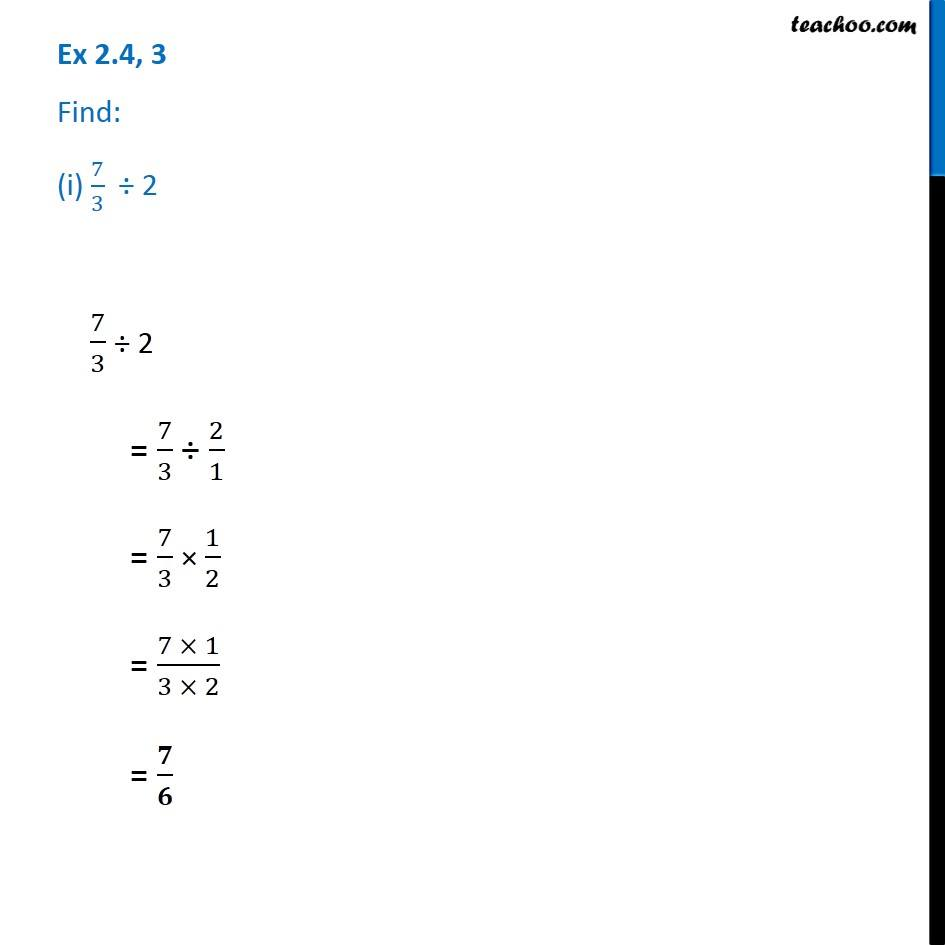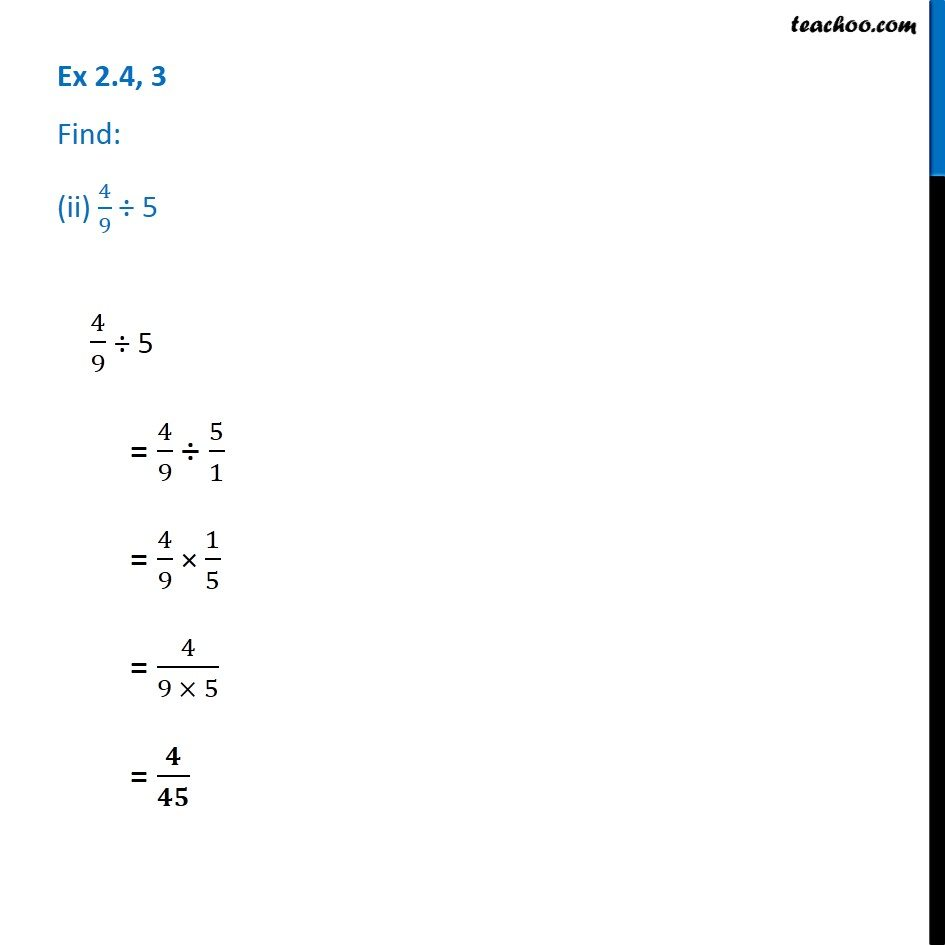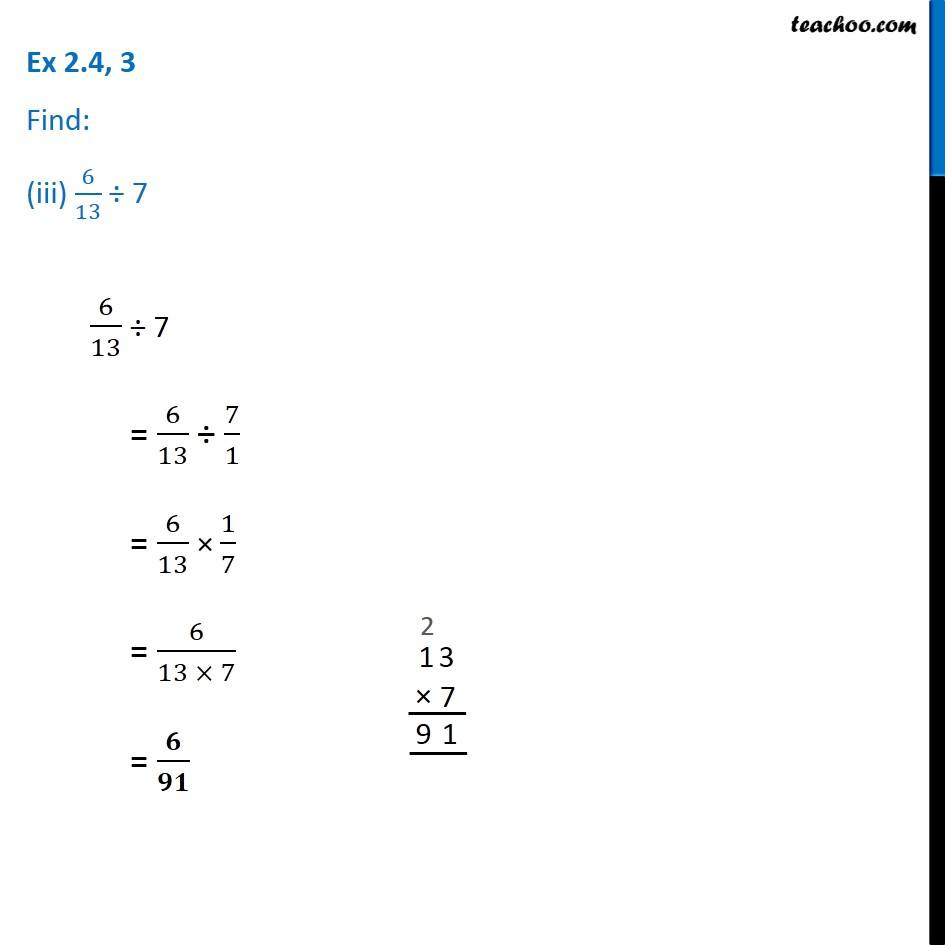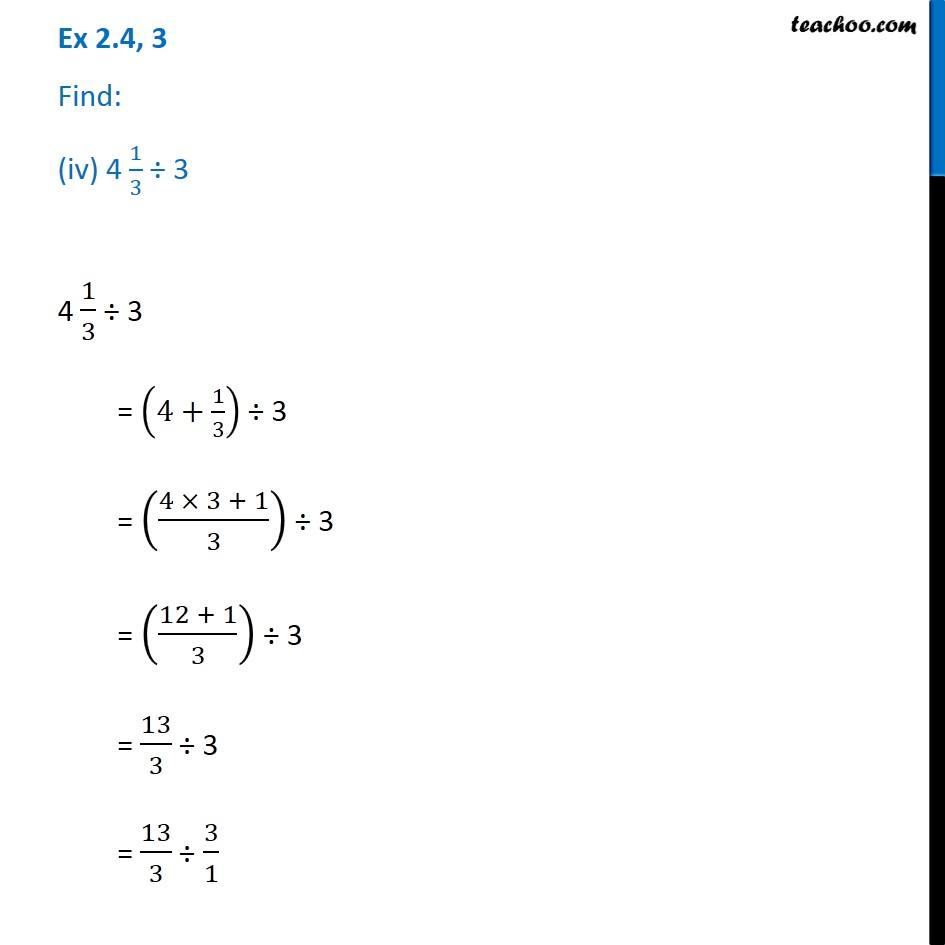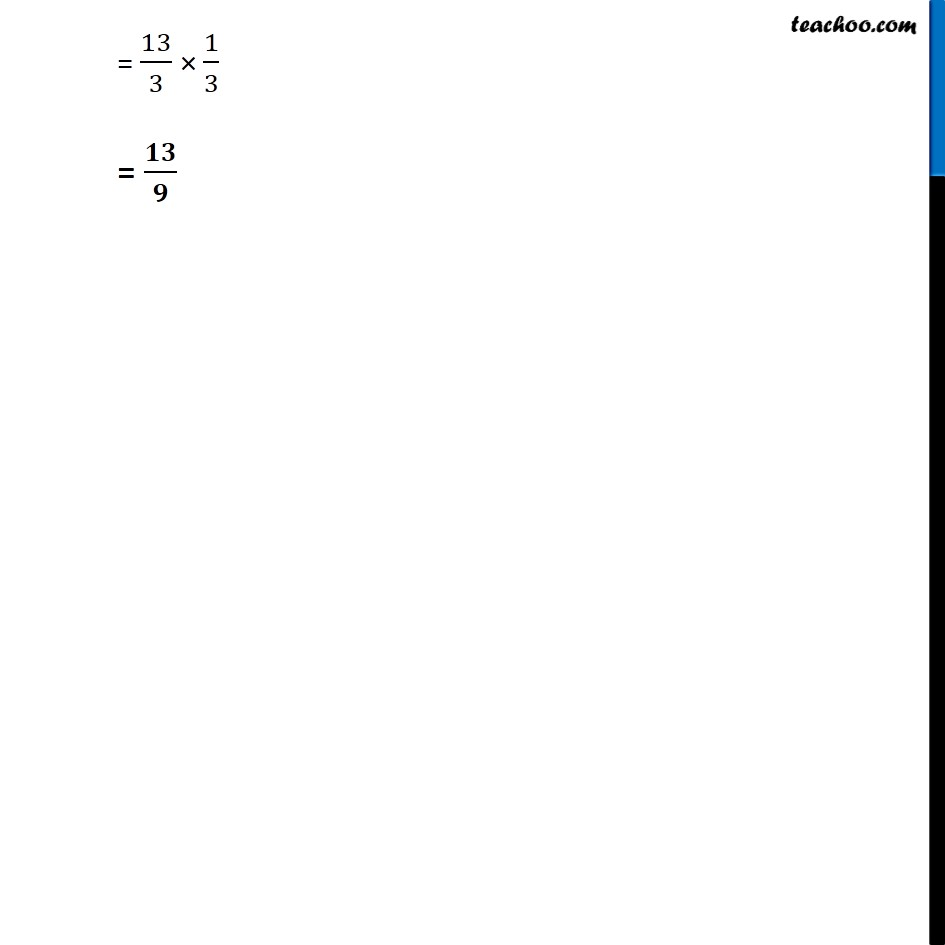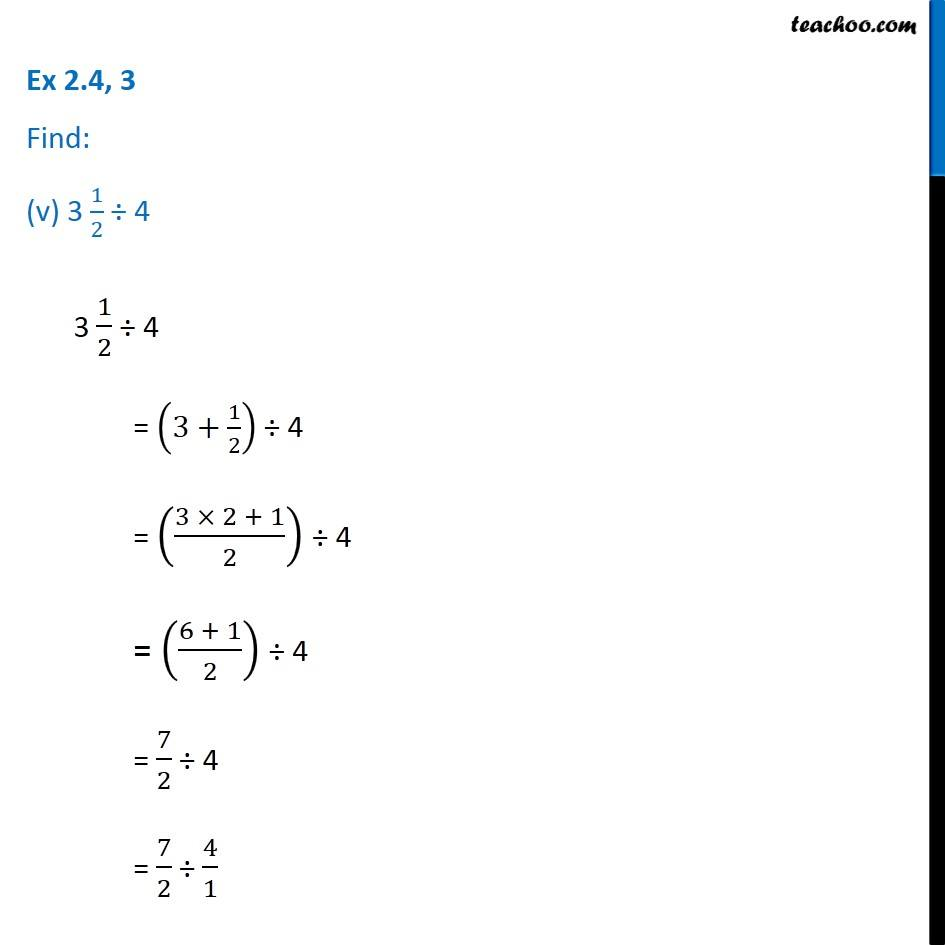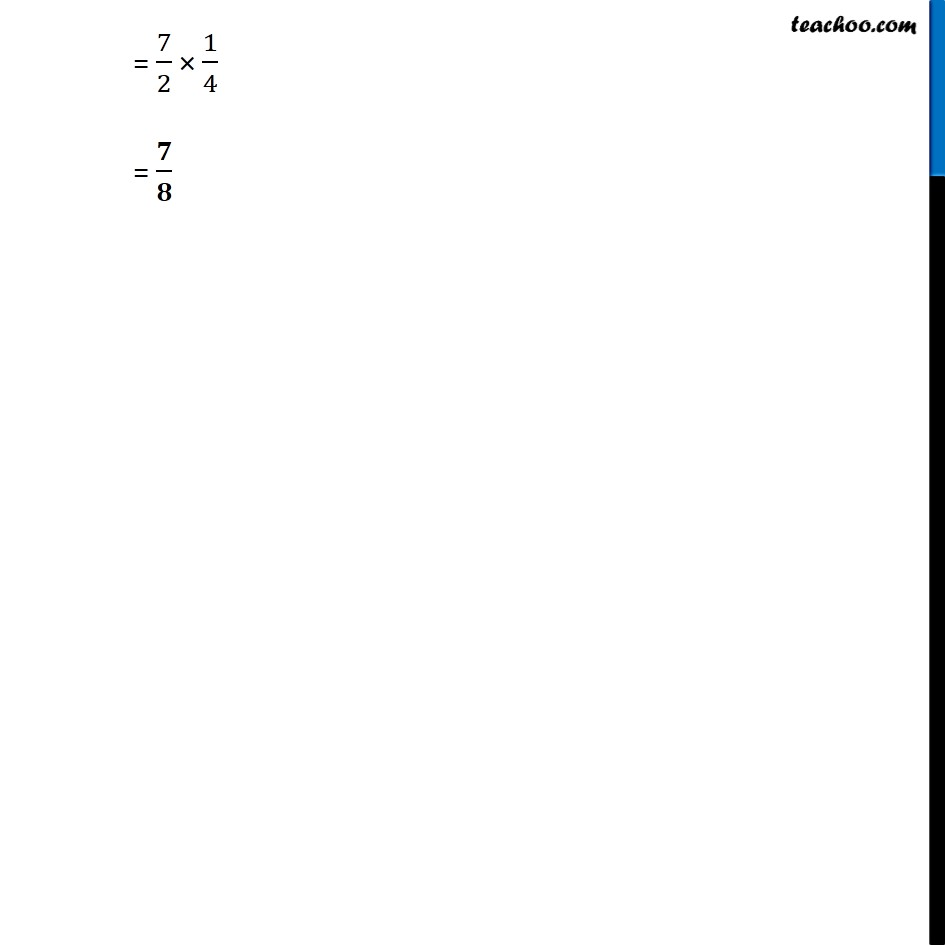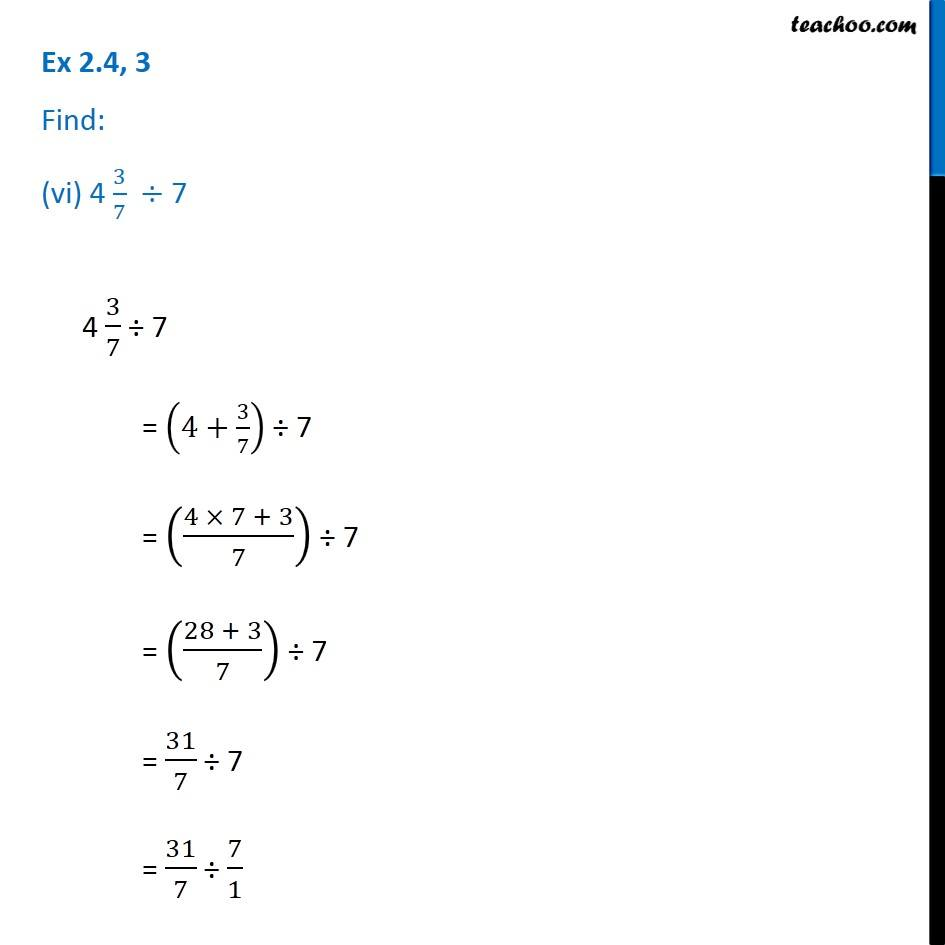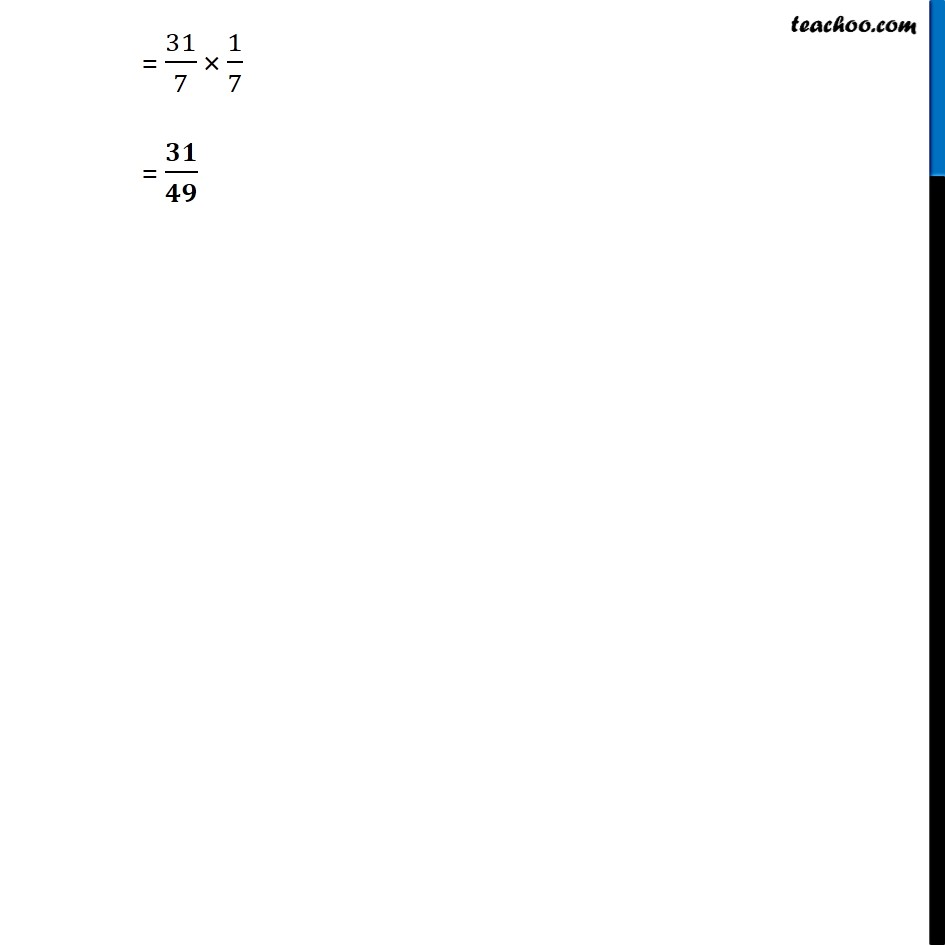1. Chapter 2 Class 7 Fractions and Decimals
2. Serial order wise
3. Ex 2.4

Transcript

Ex 2.4, 3 Find: (i) 7/3 ÷ 2 7/3 ÷ 2 = 7/3 ÷ 2/1 = 7/3 × 1/2 = (7 × 1)/(3 × 2) = 𝟕/𝟔 Ex 2.4, 3 Find: (ii) 4/9 ÷ 5 4/9 ÷ 5 = 4/9 ÷ 5/1 = 4/9 × 1/5 = 4/(9 × 5) = 𝟒/𝟒𝟓 Ex 2.4, 3 Find: (iii) 6/13 ÷ 7 6/13 ÷ 7 = 6/13 ÷ 7/1 = 6/13 × 1/7 = 6/(13 × 7) = 𝟔/𝟗𝟏 Ex 2.4, 3 Find: (iv) 4 1/3 ÷ 34 1/3 ÷ 3 = (4+1/3) ÷ 3 = ((4 × 3 + 1)/3) ÷ 3 = ((12 + 1)/3) ÷ 3 = 13/3 ÷ 3 = 13/3 ÷ 3/1 = 13/3 × 1/3 = 𝟏𝟑/𝟗 Ex 2.4, 3 Find: (v) 3 1/2 ÷ 4 3 1/2 ÷ 4 = (3+1/2) ÷ 4 = ((3 × 2 + 1)/2) ÷ 4 = ((6 + 1)/2) ÷ 4 = 7/2 ÷ 4 = 7/2 ÷ 4/1 = 7/2 × 1/4 = 𝟕/𝟖 Ex 2.4, 3 Find: (vi) 4 3/7 ÷ 74 3/7 ÷ 7 = (4+3/7) ÷ 7 = ((4 × 7 + 3)/7) ÷ 7 = ((28 + 3)/7) ÷ 7 = 31/7 ÷ 7 = 31/7 ÷ 7/1 = 31/7 × 1/7 = 𝟑𝟏/𝟒𝟗

Ex 2.4

About the AuthorDavneet Singh
Davneet Singh is a graduate from Indian Institute of Technology, Kanpur. He has been teaching from the past 10 years. He provides courses for Maths and Science at Teachoo.